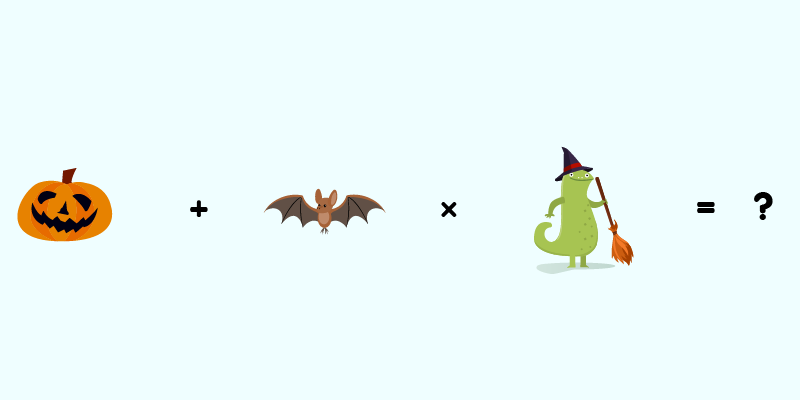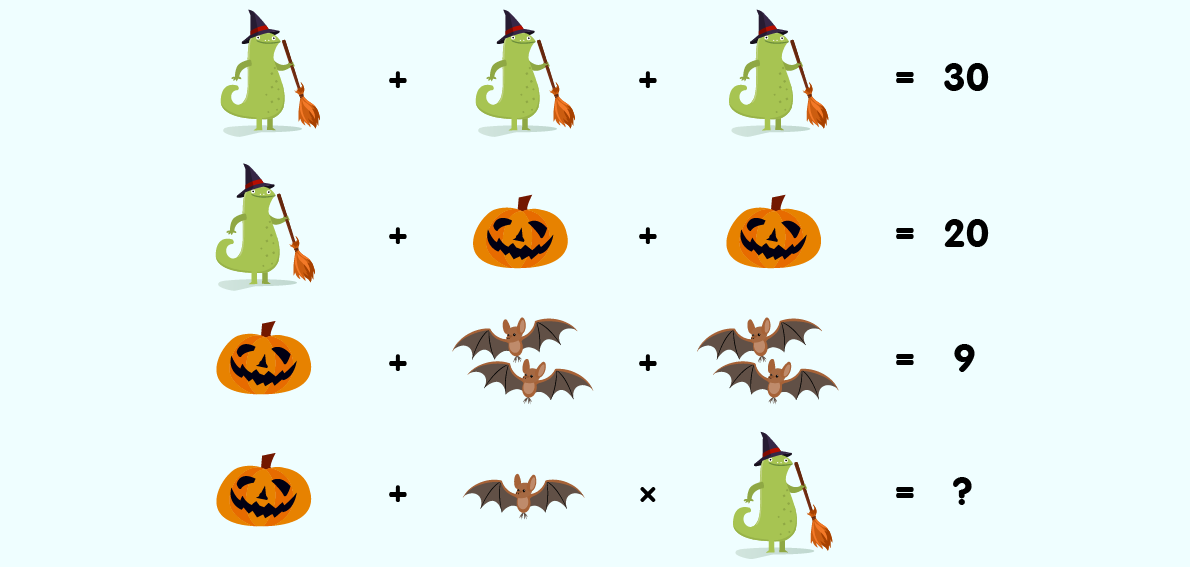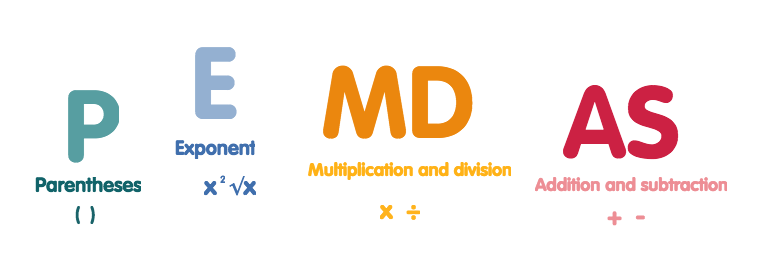# Get the answer to our tricky Halloween math puzzle!

So, how did you do?

We posed this tricky Halloween puzzle on social media:And lots of you had a try at working out the answer - but did you get it right?

...

15

Ta-da!

But how?

First, we can figure out that Komodo = 10 by dividing the answer, 30, into three equal amounts, which gives us 10.

30 ÷ 3 = 10

With the second equation, knowing that a Komodo is 10, we can take 10 from the answer to tell us what the pumpkins are together.

20 - 10 = 10

...so two pumpkins =10, making one pumpkin = 5.

We move on to the third equation and can find out the value of the groups of bats in the same way. Take the pumpkin (5) away from the answer (9) to get 4.

9 - 5 = 4

Two groups of bats = 4, so one group of bats is 2.

For the last equation, we know all the values - but we need to look carefully because there is only one bat in this equation. If a group of two bats = 2, then one bat = 1.

Which means that the final calculation we need to do is:

5 + 1 x 10 =

And this is the part where we use the order of operations law, or PEDMAS, which means that we multiply numbers before adding them. So we do the calculation 1 x 10 first, to get 10 then add this to 5 to get 15.

If you got 60, you did the adding first, which is an easy mistake to make!

Here's a little graphic to tell you a bit more about the order of calculations, or what PEDMAS stands for:Hope you enjoyed our little puzzle - you won't get caught out next time!

About Komodo – Komodo is a fun and effective way to boost K-5 math skills. Designed for 5 to 11-year-olds to use in the home, Komodo uses a little and often approach to learning math (15 minutes, three to five times per week) that fits into the busy family routine. Komodo helps users develop fluency and confidence in math – without keeping them at the screen for long.

Find out more about Komodo and how it helps thousands of children each year do better at math – you can even try Komodo for free.

And now we've got Komodo English too - check it out here.

## Related Posts

### How Wordle Can Help with Math Anxiety

Wordle, which you might think is simply a word game, is actually a mathematical puzzle. Yes, it may use words and letters instead of numbers, and a wide vocabulary doesn't hurt, but the logic, strategy, probability and elimination processes we use to solve it are actually math skills.

### Favourite story books for introducing kids to math

Children's story books are not just good for developing reading and literacy - they can help foster a good understanding of math